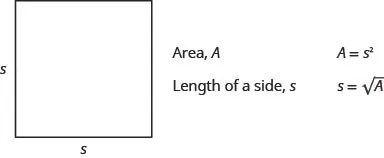Elementary Algebra

# Key Concepts

Elementary AlgebraKey Concepts

### Key Concepts

#### 9.1Simplify and Use Square Roots

• Note that the square root of a negative number is not a real number.
• Every positive number has two square roots, one positive and one negative. The positive square root of a positive number is the principal square root.
• We can estimate square roots using nearby perfect squares.
• We can approximate square roots using a calculator.
• When we use the radical sign to take the square root of a variable expression, we should specify that $x≥0x≥0$ to make sure we get the principal square root.

#### 9.2Simplify Square Roots

• Simplified Square Root $aa$ is considered simplified if $aa$ has no perfect-square factors.
• Product Property of Square Roots If a, b are non-negative real numbers, then
$ab=a·bab=a·b$
• Simplify a Square Root Using the Product Property To simplify a square root using the Product Property:
1. Step 1. Find the largest perfect square factor of the radicand. Rewrite the radicand as a product using the perfect square factor.
2. Step 2. Use the product rule to rewrite the radical as the product of two radicals.
3. Step 3. Simplify the square root of the perfect square.
• Quotient Property of Square Roots If a, b are non-negative real numbers and $b≠0b≠0$, then
$ab=abab=ab$

• Simplify a Square Root Using the Quotient Property To simplify a square root using the Quotient Property:
1. Step 1. Simplify the fraction in the radicand, if possible.
2. Step 2. Use the Quotient Rule to rewrite the radical as the quotient of two radicals.
3. Step 3. Simplify the radicals in the numerator and the denominator.

#### 9.3Add and Subtract Square Roots

• To add or subtract like square roots, add or subtract the coefficients and keep the like square root.
• Sometimes when we have to add or subtract square roots that do not appear to have like radicals, we find like radicals after simplifying the square roots.

#### 9.4Multiply Square Roots

• Product Property of Square Roots If a, b are nonnegative real numbers, then
$ab=a·banda·b=abab=a·banda·b=ab$
• Special formulas for multiplying binomials and conjugates:
$(a+b)2=a2+2ab+b2(a−b)(a+b)=a2−b2(a−b)2=a2−2ab+b2(a+b)2=a2+2ab+b2(a−b)(a+b)=a2−b2(a−b)2=a2−2ab+b2$
• The FOIL method can be used to multiply binomials containing radicals.

#### 9.5Divide Square Roots

• Quotient Property of Square Roots
• If a, b are non-negative real numbers and $b≠0b≠0$, then
$ab=abandab=abab=abandab=ab$
• Simplified Square Roots
A square root is considered simplified if there are
• no perfect square factors in the radicand
• no fractions in the radicand
• no square roots in the denominator of a fraction

#### 9.6Solve Equations with Square Roots

• To Solve a Radical Equation:
1. Step 1. Isolate the radical on one side of the equation.
2. Step 2. Square both sides of the equation.
3. Step 3. Solve the new equation.
4. Step 4. Check the answer. Some solutions obtained may not work in the original equation.
• Solving Applications with Formulas
1. Step 1. Read the problem and make sure all the words and ideas are understood. When appropriate, draw a figure and label it with the given information.
2. Step 2. Identify what we are looking for.
3. Step 3. Name what we are looking for by choosing a variable to represent it.
4. Step 4. Translate into an equation by writing the appropriate formula or model for the situation. Substitute in the given information.
5. Step 5. Solve the equation using good algebra techniques.
6. Step 6. Check the answer in the problem and make sure it makes sense.
7. Step 7. Answer the question with a complete sentence.
• Area of a Square• Falling Objects
• On Earth, if an object is dropped from a height of $hh$ feet, the time in seconds it will take to reach the ground is found by using the formula $t=h4t=h4$.
• Skid Marks and Speed of a Car
• If the length of the skid marks is d feet, then the speed, s, of the car before the brakes were applied can be found by using the formula $s=24ds=24d$.

#### 9.7Higher Roots

• Properties of
• $anan$ when $nn$ is an even number and
• $a≥0a≥0$, then $anan$ is a real number
• $a<0a<0$, then $anan$ is not a real number
• When $nn$ is an odd number, $anan$ is a real number for all values of a.
• For any integer $n≥2n≥2$, when n is odd $ann=aann=a$
• For any integer $n≥2n≥2$, when n is even $ann=|a|ann=|a|$
• $anan$ is considered simplified if a has no factors of $mnmn$.
• Product Property of nth Roots
$abn=an·bnandan·bn=abnabn=an·bnandan·bn=abn$
• Quotient Property of nth Roots
$abn=anbnandanbn=abnabn=anbnandanbn=abn$
• To combine like radicals, simply add or subtract the coefficients while keeping the radical the same.

#### 9.8Rational Exponents

• Summary of Exponent Properties
• If $a,ba,b$ are real numbers and $m,nm,n$ are rational numbers, then
• Product Property $am·an=am+nam·an=am+n$
• Power Property $(am)n=am·n(am)n=am·n$
• Product to a Power $(ab)m=ambm(ab)m=ambm$
• Quotient Property:
$aman=am−n,a≠0,m>naman=am−n,a≠0,m>n$
$aman=1an−m,a≠0,n>maman=1an−m,a≠0,n>m$
• Zero Exponent Definition $a0=1a0=1$, $a≠0a≠0$
• Quotient to a Power Property $(ab)m=ambm,b≠0(ab)m=ambm,b≠0$
Order a print copy

As an Amazon Associate we earn from qualifying purchases.